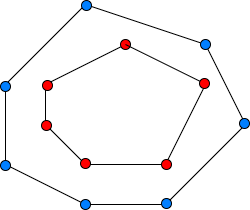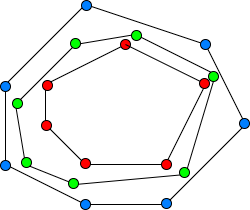# CONSEPHULLTasdidReplay of Cefalo SUST Int...
Limits 1s, 512 MB

You are given two convex polygons made out of lattice points (integer coordinates). One of them is completely inside the other one. You have to create another convex polygon that separates the two. The intermediate convex polygon may contain real coordinates but should not contain more than 2 digits after the decimal point.

In other words, build a convex polygon that is completely inside the outer convex polygon and completely outside the inner convex polygon with fulfilling the above constraints. See the figures for a better understanding.Please, refer to the note section for the definition of a convex polygon and what it means to be strictly inside of a convex polygon.

## Input

The first line contains two integers $n$ and $m$ where, $3 \leq n, \, m \leq 30.$

The following $n$ lines each contain two integers $x$ and $y$ representing a point of the outer convex polygon.

Then, the following $m$ lines each contain two integers $x$ and $y$ representing a point of the inner convex polygon.

Each point coordinate range is $0 \leq x, y \leq 10^4$.

Points will be given in a counter-clockwise order for each convex polygon.

## Output

Print an integer $p$ representing the number of points in the new convex polygon. $p$ must be at least $3$ and not greater than $1000$.

In the following $p$ lines, print the points in counter-clockwise order, forming a valid convex polygon, fulfilling all the requirements in the statement.

Note that, output containing more than 2 digits after the decimal point will receive a wrong answer verdict.

## Sample

InputOutput
4 4
0 0
10 0
10 10
0 10
5 1
9 5
5 9
1 5

4
0.56 0.65
9.35 0.85
9.64 9.00
0.8 9.8


• A convex polygon is a simple polygon where all its interior angles are strictly less than 180 degrees.

• A convex polygon is considered completely inside of another convex polygon, when each and every point of the inner polygon is strictly inside of the outer convex polygon. Points on the border of the edges are not considered as strictly inside.ConvexHull

### Submit